# Joint distribution

A general term referring to the distribution of several random variables defined on the same probability space. Letbe random variables defined on a probability space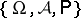and taking values in measurable spaces (cf. Measurable space)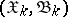. The joint distribution of these variables is the function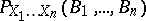of sets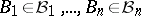, defined byIfare ordinary real random variables, then their joint distribution is the distribution of the random vector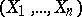in-dimensional Euclidean space(see Multi-dimensional distribution). If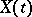,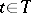, is a stochastic process, then the joint distributions of the variables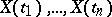for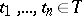are called the finite-dimensional distributions of the stochastic process.